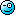## Physics Question - Shooting StarsSubmitted by reed on Sat, 2012-02-18 21:21

If I want to shoot a laser at a star that is, say, 7 light years away where do I aim so that the laser beam actually hits the star?

Do I aim at:
(1) the star I see (an image supposedly of where the star was 7 years ago); or
(2) where the star is now; or
(3) where the star will be 7 years from now?

( categories: )

### The most correct answer is...(4) None of the above.

The direction to shoot is largely dependent on the direction that the laser is traveling.

Consider the following... it is possible for two lasers to be fired from the same place at the same time in different directions and hit the same target.

### This guy didn't know what he was talking about...A laser aimed at a star that is seven light years away is no different than diagrams 2 and 3 - except perhaps that the distance from source to target has changed.

Therefore direction 2 (where the star is now) and direction 3 (where the star will be 7 years from now) are the same as direction 1 (the image of where the star was 7 years ago).

Both of the above statements are incorrect except for specific instances. The statements are true only when the extrapolated target and source paths collide or are parallel.

### Therefore...... direction 2 (where the star is now) and direction 3 (where the star will be 7 years from now) are the same as direction 1 (the image of where the star was 7 years ago).

### ExplanationFor the sake of explanation I am changing the scenario slightly - in this modified scenario I have a moving source and a moving target (star). They are moving through space in the same direction at the same speed.

Diagram 1
In this diagram I am illustrating what would happen if classical physics were correct.

In this diagram both the laser source and the target are moving. The laser beam is treated as a wave in a static medium. Under the classical physics paradigm a laser fired directly at a moving star would miss.Diagram 2
The following diagram is what happens according to the relativistic paradigm. I believe this has been experimentally verified but I couldn't find the details of the experiment.Diagram 3
The following diagram is a relativistic view of Diagram 2.Notice that when the laser light arrives at its destination in Diagrams 2 and 3 that the actual source and the apparent source are the same.

A laser aimed at a star that is seven light years away is no different than diagrams 2 and 3 - except perhaps that the distance from source to target has changed.

The relative position and movement of other stars (i.e. the rest of the universe) is irrelevant.

### Surely you'd just shoot andSurely you'd just shoot and pray, ReedWhat have you got against that star? Is it any one in particular?

### It's all relative...I have reached the conclusion that to shoot the star with a laser you have to aim at where the star appears to be in the sky, and that the apparent location is where the star actually is from our space/time frame of reference.

Feel free to disagree.

I'll do an explanation later.

It's a shame Darren has been expelled. I'm sure he'd have something to say.

### reedBy the way, this reminds me of a related problem involving time.

According to general relativity, clocks run more slowly in the vicinity of strong gravitational fields; i.e., a unit of time itself is "dilated", compared to what it would be in the absence of a gravitational field.

Relativity also tells us that "frames of reference" are all arbitrary: one is chosen over another for reasons of convenience, not because one frame of reference is objectively "more real" than any other frame of reference.

So, when calculating the apparent "age of the entire universe," where, precisely, is this supposed measurement being taken from? If the measurement assumes "empty space" with no gravitational fields, it will yield one answer; if the measurement assumes a strong gravitational field, it will yield a very different answer.

When astrophysicists tell us that the age of the universe is about 12 billions years, FROM WHERE is this measurement supposedly being taken? Near the surface of a primordial black hole? In supposedly primordial empty space? It obviously makes a difference to the final number.

### reedYou don't even need to assume a star that's 7 light years distant. The sun is 8 light-minutes away. If you wanted to hit the sun with a laser, where would you aim it? Obviously, not at where you actually observe the sun, because that's where the sun WAS 8 minutes ago.

### Richard GAre you sure?

I thought time was supposed to stand still from the photons' perspective.

### ReedAim at where the star will be 7 years from now.

### You have to aim at a pointYou have to aim at a point where the star and the laser will meet, which perhaps means none of the above. It depends on a lot of factors and variables that you have left unstated.

### I don't know the answer.The point is to gain understanding.

### What's the point of thisWhat's the point of this exercise?